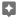# See the sum & average

You can quickly calculate the sum, average, and count in Google Sheets.

Note: This feature doesn’t work for some numbers or currency formats.

2. Highlight the cells you want to calculate.
3. In the bottom right, find Explore. Next to Explore, you'll see "Sum: total."
4. To see more calculations, click Sum.
• Average
• Minimum
• Maximum
• Count
• Count numbers
See an example
A B
2 Student 1 90%
3 Student 2 88%
4 Student 3 75%
5 Student 4 95%